DAY 13
2

Python中的多行程 (Multi-Process)

Unix 和 Linux 作業系統上提供了 fork() 系統調用來創建行程 (process)，調用 fork() 函數的是父行程 (process)，創建出的是子行程 (process)，子行程 (process)是父行程 (process) 的一個拷貝，但是子行程 (process) 擁有自己的 PID。
fork() 函數非常特殊它會返回兩次，父行程 (process) 中可以通過 fork() 函數的返回值得到子行程 (process) 的 PID，而子行程 (process) 中的返回值永遠都是 0。
Python 的 os 模組提供了 fork() 函數。由於 Windows 系統沒有 fork() 調用，因此要實現跨平台的多行程 (Multi-Process) 編程，可以使用 multiprocessing 模組的 Process 類別來創建子行程 (process)，而且該模組還提供了更高級的封裝，例如批量啟動行程的行程池 (Pool)、用於行程間通信的佇列 (Queue) 和管道 (Pipe) 等。

from random import randint
from time import time, sleep

print('開始下載 %s...' % filename)

def main():
start = time()
end = time()
print('總共耗費了 %.2f 秒.' % (end - start))

if __name__ == '__main__':
main()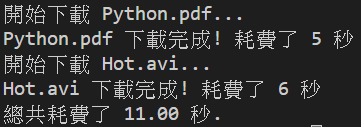from multiprocessing import Process
from os import getpid
from random import randint
from time import time, sleep

print('啟動下載行程，行程號[ %d ].' % getpid())
print('開始下載 %s...' % filename)

def main():
start = time()
# 利用 Process 類別創建了行程物件
# 透過 target 參數傳入一個函數來表示進程啟動後要執行的程式碼
# 後面的 args 代表傳遞給函數的參數
# Process 物件的 start 方法 (method) 用來啟動行程 (process)
# 而 join 方法 (method) 表示等待行程 (process) 執行結束。

p1.start()
p2.start()
p1.join()
p2.join()
end = time()
print('總共耗費了 %.2f 秒.' % (end - start))

if __name__ == '__main__':
main()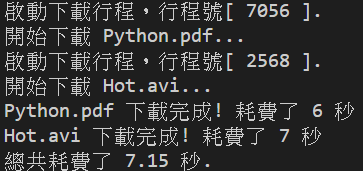from random import randint
from time import time, sleep

print('開始下載 %s...' % filename)

def main():
start = time()
t1.start()
t2.start()
t1.join()
t2.join()
end = time()
print('總共耗費了 %.3f 秒.' % (end - start))

if __name__ == '__main__':
main()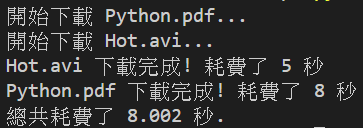from random import randint
from time import time, sleep

def __init__(self, filename):
super().__init__()
self._filename = filename

def run(self):
print('開始下載 %s...' % self._filename)

def main():
start = time()
t1.start()
t2.start()
t1.join()
t2.join()
end = time()
print('總共耗費了 %.3f 秒.' % (end - start))

if __name__ == '__main__':
main()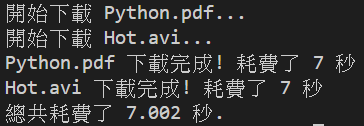from time import sleep

class Account(object):

def __init__(self):
self._balance = 0

def deposit (self, money):

new_balance = self._balance + money  # 存款後餘額
sleep(0.01)     # 模擬受理存款需要 0.01 秒的時間
self._balance = new_balance     # 修改帳戶餘額

@property
def balance(self):
return self._balance

def __init__ (self, account, money):
super().__init__()
self._account = account
self._money = money

def run(self):
self._account.deposit(self._money)

def main():
account = Account()
# 創建 100 個存款的線程 (thread) 向同一個帳戶中存錢
for _ in range(100):
t.start()
t.join()
print('帳戶餘額為 : %d 元' % account.balance)

if __name__ == '__main__':
main()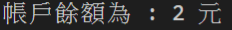from time import sleep

class Account(object):

def __init__(self):
self._balance = 0
self._lock = Lock()

def deposit (self, money):
# 先獲取所才能執行後續後續的程式碼
self._lock.acquire()
try:
new_balance = self._balance + money
sleep(0.01)
self._balance = new_balance
finally:
# 在 finally 中執行釋放鎖保證正常異常鎖都能釋放
self._lock.release()

@property
def balance(self):
return self._balance

def __init__ (self, account, money):
super().__init__()
self._account = account
self._money = money

def run(self):
self._account.deposit(self._money)

def main():
account = Account()
for _ in range(100):
t.start()
t.join()
print('帳戶餘額為 : %d 元' % account.balance)

if __name__ == '__main__':
main()30天學python30